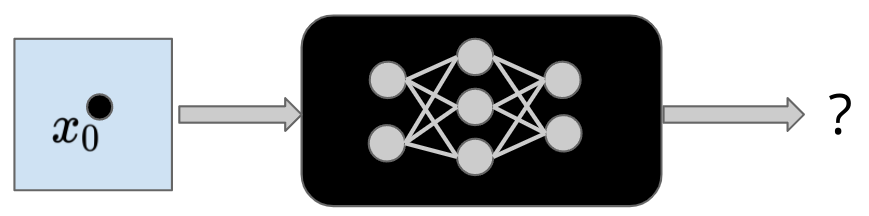# Formal Verification of Deep Neural Networks: Theory and PracticeA introductory and hands-on tutorial for neural network verification, including both basic mathematical background and coding examples.A tutorial presented by Huan Zhang, Kaidi Xu, Shiqi Wang and Cho-Jui Hsieh

# Introduction

Neural networks have become a crucial element in modern artificial intelligence. However, they are often black-boxes and can behave unexpectedly and produce surprisingly wrong results, such as adversarial examples. In this tutorial, we introduce the neural network verification problem, which aims to formally guarantee properties of neural networks such as robustness, safety, and correctness. Our tutorial covers the theoretical foundations of the verification problem and an introduction to state-of-the-art algorithms. Furthermore, we will give a hands-on coding tutorial for user-friendly neural network verification toolboxes, allowing practitioners to easily apply formal verification techniques to their customized applications.

Our tutorial includes coding demonstrations in Google Colab. We will demonstrate the usage of the versatile auto_LiRPA library and the award-winning α,β-CROWN verifier.

# Outline

In the first half of the tutorial, we introduce Mathematical Backgrounds and Algorithms for neural network verification, including problem formulation, incomplete verification algorithms (with a focus on state-of-the-art bound propagation based algorithms such as CROWN), complete verification algorithms (e.g., integer programming formulation and branch and bound based methods). The second half is a Hands-on Tutorial with interactive coding examples to help the participants understand the verification problem and apply state-of-the-art neural network verification tools to their own applications. Coding examples include adversarial robustness verification problem on a CIFAR-10 classifier and a certified adversarial defense for a natural language model (LSTM/Transformer). Finally, we demonstrate how to use the state-of-the-art and award-winning complete neural network verifier α,β-CROWN (also written as alpha-beta-CROWN).

# Slides

The slides for each part of the tutorial are provided below:

Part I: Introduction to Neural Network Verifier

Part II: Neural Network Verification Algorithms

Part III: Hands-on Tutorial on Using State-of-the-art Verification Tools

# Coding Demos:

Colab Demo for the auto_LiRPA library.

Colab Demo for the α,β-CROWN (alpha-beta-CROWN) library.

# Related Videos

Here are some relevant pre-recorded videos on neural network verification by the tutorial organizers.

AAAI 2022 Tutorial: “Formal Verification of Deep Neural Networks: Theory and Practice”

CMU AI Seminar: “How We Trust a Black-box: Formal Verification of Deep Neural Networks”

A Short Tutorial on Auto_LiRPA: An Automatic Library for Neural Network Verification and Scalable Certified Defense

*****

A neural network verification tutorial presented by Huan Zhang, Kaidi Xu, Shiqi Wang and Cho-Jui Hsieh

☕ Pudhina theme by Knhash 🛠️